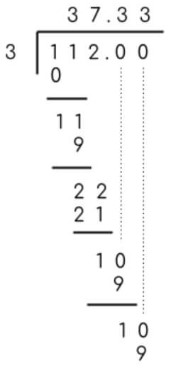# Long Division Calculator With Remainders

To find the quotient and remainder, enter the divisor & dividend, and press the calculate button using long division calculator

 divisor dividend

Give Us Feedback

## Long Division Calculator with steps

Long division calculator is used for problems regarding division operation. It has two main functions. It can solve

1. Long division with remainder
2. Long division with decimal

The tool is user-friendly and can be used without any previous experience. The result consists of two parts; the end result and the step-by-step long division.

You can choose the number of decimals as well. If you like this tool then make sure to visit Long Addition Calculator as well. Let’s learn about long division and the methods used to solve it.

## How to use long division calculator?

To use the long division calculator, you will have to

1. Choose the division type i.e with a remainder or decimal answer.
2. Enter the value of dividend and divisor.
3. Click Calculate.

The result will show right after you complete these steps.

## What is Long division?

The process of solving the division process in multiple steps is called long division. The dividend and divider usually consist of 2 or more digits.

In short, it is the division method that is detailed. Now, it is further divided into two types.

### Long Division with the remainder:

It is the process in which the dividend is Divided up to only the whole quotient. If the dividend becomes smaller than the divisor it is left untouched at the bottom.

This left number is known as the remainder. To solve questions with this method you have to solve the dividend until no more division is possible.

Let’s see an example for a better understanding.

Example:

Divide 234 by 5

Solution:

Step 1: Write 234 under the long division symbol.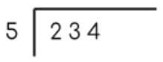Step 2: Make the first divisible pair since 2 is smaller than 5.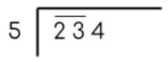Step 3: Solve.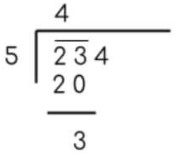Step 4: Bring down 4 to make a divisible number.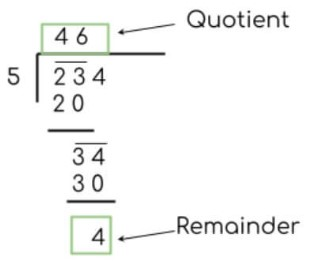Hence 4 is the remainder.

### Long division with decimal:

There are two main reasons to use this method.

1. Your dividend or divisor has a decimal point.
2. You want a quotient in decimal value.

If the divisor is in decimal value, the decimal point is moved in such a way to make it a whole number. To balance this step, the decimal point is also moved in the dividend value.

Example:

Solve for three decimal places:

Divisor: 2.1

Dividend: 346

Solution:

Step 1: Move the decimal point one place to the right in the divisor.

Divisor = 21.0

Since the divisor is changed, the dividend will also need changing.

Dividend = 3.46

Step 2: Solve.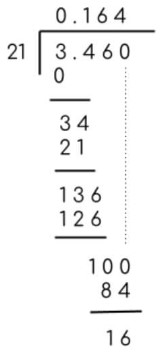If both dividend and divisor are in whole numbers and you want a decimal value quotient, then you add a point in the quotient and zero with the remainder to continue solving.

Example:

Solve 112/3 for 2 decimal values.

Solution: